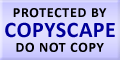Submit Assignment From Here
Name
:
Email
:
Phone
:
Country
:
Willing to pay US\$
:
Subject
:
Topic
:
Levels
:
:Hours
:

# Statistics Homework Help

## Help on Statistics Homework and Assignments

If you are looking for Statistics homework help or Statistics Assignment Help then its worth giving us a chance to serve you. In Simple language Statistics is collecting the data, organizing it and then analysing the data to make comparisons. Statistics is a part of applied mathematics. Modern Statistics also includes the designing analysing the surveys, quantification of social and scientific aspects along with the principles of stats to understand the world around us. Because of the data being used now days in most of the areas of human workings, theories and methods of advanced Statistics have been applied mostly everywhere.

### Statistics include

1. Descriptive Statistics: Descriptive Statistics means analysing the data to describe it in meaningful way. However, we cannot have a conclusion depending on the descriptive data. Descriptive data further include:
1. Measure of central tendency
2. Inferential Statistics: Inferential Statistics is the next process that comes after Descriptive Statistics. With the help of Inferential Statistics, we reach conclusion extending the immediate data alone. We use Inferential Statistics to have judgements or figuring out the end results or conclusions. Inferential Statistics include
1. Experimental Analysis
2. Quasi- Experimental Analysis

### Statistics Homework Help at Assignment Hut

Do my Statistics Homework

Statistics problems were the central idea for developing Assignment Hut online tutoring. The tangled problems, its correlation and finding of measure of central tendency made Assignment Hut exist. As finding out the solutions and understanding these concepts in such an easy way was not an option. These problems made us also look for solutions in books which was so time consuming and confusing. Being the victim of unsolved statistics problems, statistics help via Assignment Hut came into existence

We understand how students go through the roller coaster of solving these problems, but through our statistics homework help these nightmare statistics problems no longer exist. Our online tutors will be available even to solve your single question query. If students face any problem in understanding the solution they can further get back to our tutors and in simpler way solution will be given according to the priority of the student through Statistics homework help.

Stream specific Students may refer Statistics Online TutoringUS